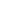Optical square is:

a) Engineer’s square having stock and blade set at 90°
b) A constant deviation prism having the angle of deviation between the incident ray and reflected ray, equal to 90°
c)  A constant deviation prism having the angle of deviation between the incident ray and reflected ray, equal to 45°
d) Used to produce interference fringes
ISRO Scientist-2010

 A constant deviation prism having the angle of deviation between the incident ray and reflected ray, equal to 45°.
Share :
+
Next
« Prev
Prev
Next »